## Row Space and Column Space of a Matrix

Let A be an m by n matrix. The space spanned by the rows of A is called the row space of A, denoted RS(A); it is a subspace of R n . The space spanned by the columns of A is called the column space of A, denoted CS(A); it is a subspace of R m .

The collection { r 1, r 2, …, r m } consisting of the rows of A may not form a basis for RS(A), because the collection may not be linearly independent. However, a maximal linearly independent subset of { r 1, r 2, …, r m } does give a basis for the row space. Since the maximum number of linearly independent rows of A is equal to the rank of A,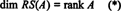Similarly, if c 1, c 2, …, c n denote the columns of A, then a maximal linearly independent subset of { c 1, c 2, …, c n } gives a basis for the column space of A. But the maximum number of linearly independent columns is also equal to the rank of the matrix, so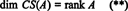Therefore, although RS(A) is a subspace of R n and CS(A) is a subspace of R m , equations (*) and (**) imply thateven if m ≠ n.

Example 1: Determine the dimension of, and a basis for, the row space of the matrix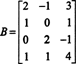A sequence of elementary row operations reduces this matrix to the echelon matrix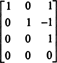The rank of B is 3, so dim RS(B) = 3. A basis for RS(B) consists of the nonzero rows in the reduced matrix: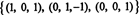Another basis for RS(B), one consisting of some of the original rows of B, isNote that since the row space is a 3‐dimensional subspace of R 3, it must be all of R 3.

Criteria for membership in the column space. If A is an m x n matrix and x is an n‐vector, written as a column matrix, then the product A x is equal to a linear combination of the columns of A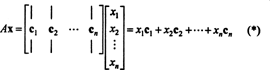By definition, a vector b in R m is in the column space of A if it can be written as a linear combination of the columns of A. That is, bCS(A) precisely when there exist scalars x 1, x 2, …, x n such thatCombining (*) and (**), then, leads to the following conclusion: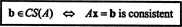Example 2: For what value of b is the vector b = (1, 2, 3, b) T in the column space of the following matrix?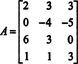Form the augmented matrix [ A/ b] and reduce: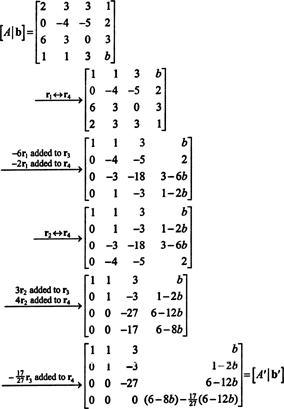Because of the bottom row of zeros in A′ (the reduced form of A), the bottom entry in the last column must also be 0—giving a complete row of zeros at the bottom of [ A′/ b′]—in order for the system A x = b to have a solution. Setting (6 − 8 b) − (17/27)(6 − 12 b) equal to 0 and solving for b yieldsTherefore, b = (1, 2, 3, b) T is in CS(A) if and only if b = 5.

Since elementary row operations do not change the rank of a matrix, it is clear that in the calculation above, rank A = rank A′ and rank [ A/ b] = rank [ A′/ b′]. (Since the bottom row of A′ consisted entirely of zeros, rank A′ = 3, implying rank A = 3 also.) With b = 5, the bottom row of [ A′/ b′] also consists entirely of zeros, giving rank [ A′/ b′] = 3. However, if b were not equal to 5, then the bottom row of [ A′/ b′] would not consist entirely of zeros, and the rank of [ A′/ b′] would have been 4, not 3. This example illustrates the following general fact: When b is in CS(A), the rank of [ A/ b] is the same as the rank of A; and, conversely, when b is not in CS(A), the rank of [ A/ b] is not the same as (it's strictly greater than) the rank of A. Therefore, an equivalent criterion for membership in the column space of a matrix reads as follows:Example 3: Determine the dimension of, and a basis for, the column space of the matrix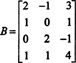from Example 1 above.

Because the dimension of the column space of a matrix always equals the dimension of its row space, CS(B) must also have dimension 3: CS(B) is a 3‐dimensional subspace of R 4. Since B contains only 3 columns, these columns must be linearly independent and therefore form a basis: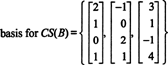Example 4: Find a basis for the column space of the matrix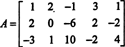Since the column space of A consists precisely of those vectors b such that A x = b is a solvable system, one way to determine a basis for CS(A) would be to first find the space of all vectors b such that A x = b is consistent, then constructing a basis for this space. However, an elementary observation suggests a simpler approach: Since the columns of A are the rows of A T, finding a basis for CS(A) is equivalent to finding a basis for RS(A T) . Row‐reducing A T yields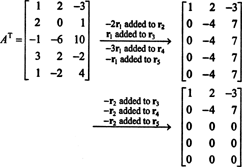Since there are two nonzero rows left in the reduced form of A T, the rank of A T is 2, soFurthermore, since { v 1, v 2} = {(1, 2, −3), (0, −4, 7)} is a basis for RS(A T), the collectionis a basis for CS(A), a 2‐dimensional subspace of R 3.